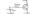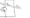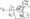# Power Electronics-1 January 2013

UNIT – I

1 (a) Consider on open circuited P-n junction. Sketch curves as a function of distance across the junction of space charge, electric field and potential.

(b)Explain zener break down. Also explain working of a zener diode.

OR

1 (a) How does the dynamic resistance ‘r’ of a diode vary with

(i)  Current and

(ii) Temperature

(iii) What is the order of magnitude of r for silicon at room temperature and for a dc current of 1mA ?

(b)Explain photo diodes. Does there is any relationship between Photo Diodes and Solar Cell ?

UNIT – II

2. (a) What are electronics filters ? Explain various types of filters with their applications.

(b) Explain single phase full wave bridge rectifiers. Can the transformer and the load be interchanged in it ?

OR

(a) Prove that the regulation of both the half wave and full wave rectifier is given by Rf % Regulation =100%

(b) A symmetrical SK kHz square wave whose output varies between + 10 and – 10V is impressed upon the clipping circuit

shown. Assume R,=0,R —2M .

UNIT -III

3. (a) Sketch the hybrid h-model for CE configuration and find the expression for rbce, Sm and rce in terms of h-parameter constant of a transistor.

(b) Find the transistor current in the circuit of fig below a silicon transistor with |3 = \ 00 and IC() — 20n A = 2x\Q~^mA is under considceration.

OR

(a) Explain concept of minority carrier concentration in the base for cutoff, active and saturation conditions in a BJT.

(b) A silicon transistor with VBE sat =0.8V, 3 = hfE = 100, VCE. sai = is used in the circuit shown. Find the minimum value of for which the transistor remain in saturation.

UNIT- IV

4. (a)Explain construction, working and V-I characteristics of a JFET.(b) Explain DC analysis of FET, also explain FET as a voltage variable resistor.

OR

(a) Explain MOSFET with its type, construction and V-I characteristics ?

(b) The amplifier shown in fig utilized an n-channel FET for which Vp = -2.0 V and Ipkss= l-65mA. It is desired to bias the circuit at ID = 0.8mA, using VDD = 24V. Assume r(|»R(| find Such that the voltage gain is at least 20 dB, with Rs bypassed with a very large capacitance Cs.UNIT -V

5. (a) Sketch the circuit for Darlington pair and find its overall current gain with help of its ac equivalent circuit.

(b) Explain frequency response of CE transistor as amplifier at high frequency.

OR

Write short notes on any two :

(a) Bootstrapped Darligton circuit

(b) Miller’s theorem and its dual